## Archive for the ‘TURBO MACHINE’ category

### Hydraulic Turbine

September 20, 2012

### Turbo-Charger

September 28, 2011A turbocharger is actually a type of supercharger. Originally, the turbocharger was called a “turbo super charger.” Obviously, the name was shortened out of convenience.A turbocharger’s purpose is to compress the oxygen entering a car’s engine, increasing the amount of oxygen that enters and thereby increasing the power output. Unlike the belt-driven supercharger that is normally thought of when one hears the word “supercharger,” the turbocharger is powered by the car’s own exhaust gases. In other words, a turbocharger takes a by-product of the engine that would otherwise be useless, and uses it to increase the car’s horsepower.Cars without a turbocharger or supercharger are called normally aspirated. Normally aspirated cars draw air into the engine through an air filter; the air then passes through a meter, which monitors and regulates the amount of air that enters the system. The air is then delivered to the engine’s combustion chambers, along with a controlled amount of fuel from the carburetor or fuel injectors.

In a turbocharged engine, however, the air is compressed so that more oxygen will fit in the combustion chamber, dramatically increasing the burning power of the engine. The turbocharger is composed of two main parts: the compressor, which compresses the air in the intake; and the turbine, which draws the exhaust gases and uses them to power the compressor. Another commonly used term in relation to turbochargers is boost, which refers to the amount of pressure the air in the intake is subjected to; in other words, the more compressed the air is, the higher the boost.

Although the increase in power is advantageous to the car — and likely a source of enjoyment for the driver — a turbocharger has its drawbacks. First and foremost, a turbocharged engine must have a lower compression ratio than a normally aspirated engine. For this reason, one cannot simply put a turbocharger on an engine that was intended for normal aspiration without seriously undermining the life and performance of the engine. Also, a lower compression ratio means the engine will run less efficiently at low power.

Another major drawback of a turbocharger is the phenomenon known as turbo lag. Because the turbocharger runs on exhaust gases, the turbine requires a build-up of exhaust before it can power the compressor; this means that the engine must pick up speed before the turbocharger can kick in. Additionally, the inlet air grows hotter as it is compressed, reducing its density, and thereby its efficiency in the combustion chamber; a radiator-like device called an intercooler is often used to counter this effect in turbocharged engines.

### Super Charger

September 28, 2011

Engines combust (burn) fuel and use the energy of that combustion to do work. The more fuel that is combusted in any given time then the more energy is available to carry out the engines task. Fuel requires air (or the oxygen contained within air) to burn so if there isn’t enough air mixed with the fuel it will not burn. This also means that the amount of air entering an engine determines how much fuel can be burnt and consequently how much energy (or power) an engine can produce. Superchargers are essentially an air pump designed to cram extra air into an engine allowing it to combust more fuel than would otherwise be possible.Mercedes pioneered automotive superchargers on their race cars during the 1920’s. These were simple reciprocating compressors attached to the engine by an electrically operated clutch. A switch activated by the accelerator pedal turned the pump on when extra power (full throttle) was required. A flurry of engineering endeavor ensued in order to reign in Mercedes advantage on the racetrack. Within a few short years most of the basic designs for modern superchargers had appeared.During the 1930’s superchargers were largely the preserve of marine engines, aircraft and race vehicles but gradually found their way into commercial diesel engines by the 1950’s. It has been common for truck engines to be turbo supercharged (a.k.a. turbocharged) for decades but car engines originally had difficulty in effectively employing this technology.Superchargers mostly fall into one of two categories, mechanically driven superchargers and turbo superchargers driven by exhaust gasses. A third category is starting to make an appearance and that is electrically powered superchargers.Turbo superchargers (a.k.a. turbochargers or turbo’s) are relatively compact, lightweight and efficient but suffer from turbo lag and heat stress. By turbo lag we mean the amount of time it takes for the turbo’s rotor to speed up to full efficiency. Some of the earliest turbo charged vehicles took so long for the turbo to produce a usable amount of boost that they were all but useless. Modern turbo chargers are much better in this regard but turbo lag is still a problem. Heat is another bane of turbo chargers. Exhaust gasses are extremely hot and can cause so much heat to build up in the turbo that oil will burn and congeal within its galleries leading to a bearing failure. This is why many turbo chargers have a turbo timer. The timer will cause an engine to continue idling for a few minutes after it is switched off allowing excess heat to be dissipated.Mechanically driven superchargers usually don’t suffer from turbo lag and can often produce more boost than an exhaust driven charger (turbo). On the negative side they are generally bulky, heavy, and have a cumbersome drive mechanism (usually belt drive). Furthermore most chargers of this type have to supply air at all engine speeds and loads making them difficult to match various engine conditions precisely.

As our supercharger is electrically driven we have devoted an entire article to the advantages and disadvantages of this type.

Heat exchangers (intercoolers) are frequently used in conjunction with superchargers. Compressing air increases its temperature thus making it less dense. By re-cooling the compressed volume of air before it enters, density is increased allowing even more air to be forced into the engine. Intercoolers are more important for turbo superchargers as there are two heating sources present, the act of compression and heat from exhaust gasses both increase air temperature.

### 12. Energy Exchange with Moving Blades

September 22, 2011

#Subsections

• 12.1 Introduction
• 12.2 Conservation of Angular Momentum
• 12.3 The Euler Turbine Equation
• 12.4 Multistage Axial Compressors
• 12.5 Velocity Triangles for an Axial Compressor Stage
• 12.6 Velocity Triangles for an Axial Flow Turbine Stage

# 12.1 Introduction

So far we have only looked at the thermodynamic results of compressors and turbines (‘s and‘s). Now we will look in more detail at how the components of a gas turbine engine produce these effects. You will learn later that without heat transfer, it is only possible to change the total enthalpy of a fluid with an unsteady process (e.g. moving blades). Still we will use many of the steady flow tools that we have discussed in thermodynamics and propulsion by considering the steady flow in and out of a component as shown in Figure 12.1.# 12.2 Conservation of Angular Momentum

[This section is excerpted from Fluid Flow: A First Course in Fluid Mechanics, Macmillan Publishing Company, 1989.]

The momentum theorem developed in Chapter 10 gives the force acting on a fixed volume in terms of linear momentum flux through the surface of the volume. In many situations we are interested in the moment or torque on the volume. For this purpose we may adapt the angular momentum law of mechanics to the flow of fluids. Our starting point is the familiar law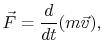where,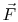, andrefer to a single particle. The torque exerted by the forceabout a fixed point iswhereis the radius vector from the fixed point to the point of application of. The symbol,, signifies, as usual, that the vector cross-product shall be taken. Then, from Newton’s law of motion,We now define a vectoras the vector product of the radius vector to the particle and the linear momentum, that is,The quantityis called angular momentum. Upon differentiatingwith respect to time, we find thatHowever,and the cross-product of a vector parallel to itself is zero. The first term in the right-hand side therefore vanishes and we have the result that(12..1)

Equation (12.1) states that the rate of change of angular momentum of a particle about a fixed point is equal to the torque applied to the particle.We now seek to modify the law as expressed by Equation (12.1) to be suitable for a fixed volume. The torque on a material volume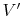is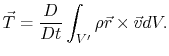This is readily transformed into a control volume integral. We have, therefore,where(12..2)

is the angular momentum contained within the control volume. Equation (12.2) represents the angular momentum theorem. [For more information about angular momentum and rotational energy, see pages 246 and 558 in Hibbeler’s Engineering Dynamics.]

# 12.3 The Euler Turbine Equation

The Euler turbine equation relates the power added to or removed from the flow, to characteristics of a rotating blade row. The equation is based on the concepts of conservation of angular momentum and conservation of energy. We will work with the model of the blade row shown in Figure 12.2.Applying conservation of angular momentum, we note that the torque,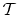, must be equal to the time rate of change of angular momentum in a streamtube that flows through the deviceThis is true whether the blade row is rotating or not. The sign matters (i.e. angular momentum is a vector – positive means it is spinning in one direction, negative means it is spinning in the other direction). So depending on how things are defined, there can be positive and negative torques, and positive and negative angular momentum. In Figure 9.2, torque is positive when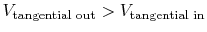V_{textrm{tangential in}}\$” width=”207″ height=”32″ align=”MIDDLE” border=”0″ /> — the same sense as the angular velocity.If the blade row is moving, then work is done on/by the fluid. The work per unit time, or power,, is the torque multiplied by the angular velocity,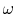:If torque and angular velocity are of like sign, work is being done on the fluid (a compressor). If torque and angular velocity are of opposite sign work is being extracted from the fluid (a turbine). Here is another approach to the same idea:

• If the tangential velocity increases across a blade row (where positive tangential velocity is defined in the same direction as the rotor motion) then work is added to the flow (this happens in a compressor).
• If the tangential velocity decreases across a blade row (where positive tangential velocity is defined in the same direction as the rotor motion) then work is removed from the flow (this happens in a turbine).

From the steady flow energy equation,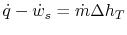with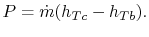Then equating this expression of conservation of energy with our expression from conservation of angular momentum, we arrive at:or for a perfect gas with,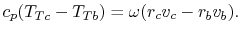(12..3)

Equation (12.3) is called the Euler Turbine Equation. It relates the temperature ratio (and hence the pressure ratio) across a turbine or compressor to the rotational speed and the change in momentum per unit mass. Note that the velocities used in this equation are what we will later call absolute frame velocities (as opposed to relative frame velocities).

• If angular momentum increases across a blade row, thenT_{Tb}\$” width=”80″ height=”32″ align=”MIDDLE” border=”0″ /> and work was done on the fluid (a compressor).
• If angular momentum decreases across a blade row, then <img src="http://web.mit.edu/16.unified/www/SPRING/propulsion/notes/img1569.png&quot; alt="\$ T_{Tc}  and work was done by the fluid (a turbine).

# 12.4 Multistage Axial Compressors

An axial compressor is typically made up of many alternating rows of rotating and stationary blades called rotors and stators, respectively, as shown in Figures 12.3 and 12.4. The first stationary row (which comes in front of the rotor) is typically called the inlet guide vanes or IGV. Each successive rotor-stator pair is called a compressor stage. Hence compressors with many blade rows are termed multistage compressors.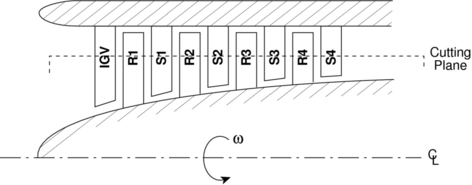One way to understand the workings of a compressor is to consider energy exchanges. We can get an approximate picture of this using the Bernoulli Equation, whereis the stagnation pressure, a measure of the total energy carried in the flow,is the static pressure, a measure of the internal energy, and the velocity terms are a measure of the kinetic energy associated with each component of velocity (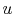is radial,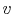is tangential,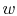is axial).The rotor adds swirl to the flow, thus increasing the total energy carried in the flow by increasing the angular momentum (adding to the kinetic energy associated with the tangential or swirl velocity,).The stator removes swirl from the flow, but it is not a moving blade row and thus cannot add any net energy to the flow. Rather, the stator rather converts the kinetic energy associated with swirl to internal energy (raising the static pressure of the flow). Thus typical velocity and pressure profiles through a multistage axial compressor look like those shown in Figure 12.5.Note that the IGV also adds no energy to the flow. It is designed to add swirl in the direction of rotor motion to lower the Mach number of the flow relative to the rotor blades, and thus improve the aerodynamic performance of the rotor.

# 12.5 Velocity Triangles for an Axial Compressor Stage

Velocity triangles are typically used to relate the flow properties and blade design parameters in the relative frame (rotating with the moving blades), to the properties in the stationary or absolute frame.

We begin by “unwrapping” the compressor. That is, we take a cutting plane at a particular radius (e.g. as shown in Figure 12.4) and unwrap it azimuthally to arrive at the diagrams shown in Figure 12.6. Here we have assumed that the area of the annulus through which the flow passes is nearly constant and the density changes are small so that the axial velocity is approximately constant.In drawing these velocity diagrams it is important to note that the flow typically leaves the trailing edges of the blades at approximately the trailing edge angle in the coordinate frame attached to the blade (i.e. relative frame for the rotor, absolute frame for the stator).

We will now write the Euler Turbine Equation in terms of stage design parameters:, the rotational speed, and, the leaving angles of the blades.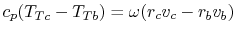From geometry,and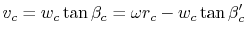soorSo we see that the total or stagnation temperature rise across the stage increases with the tip Mach number squared, and for fixed positive blade angles, decreases with increasing mass flow. This behavior is represented schematically in Figure 12.7.# 12.6 Velocity Triangles for an Axial Flow Turbine Stage

We can apply the same analysis techniques to a turbine, Figure 12.8. The stator, again does no work. It adds swirl to the flow, converting internal energy into kinetic energy. The turbine rotor then extracts work from the flow by removing the kinetic energy associated with the swirl velocity.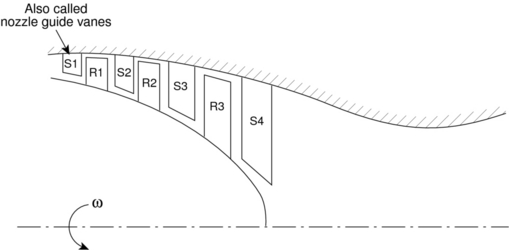The appropriate velocity triangles are shown in Figure 12.9, where again the axial velocity was assumed to be constant for purposes of illustration. As we did for the compressor, we can write the Euler Turbine Equation in terms of useful design variables: